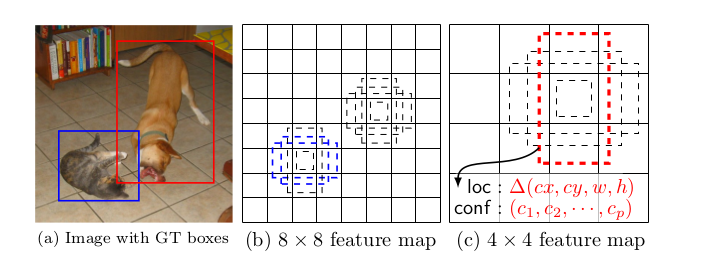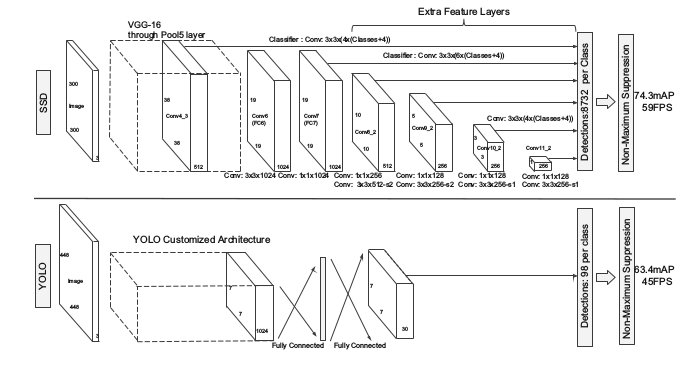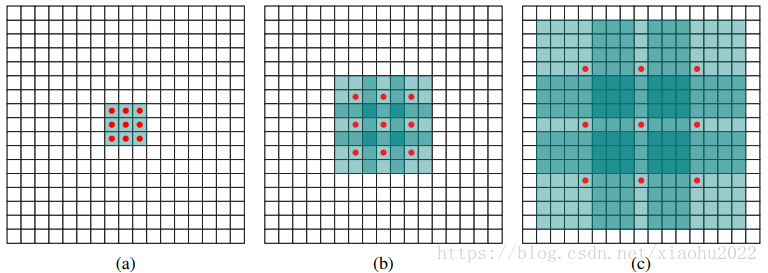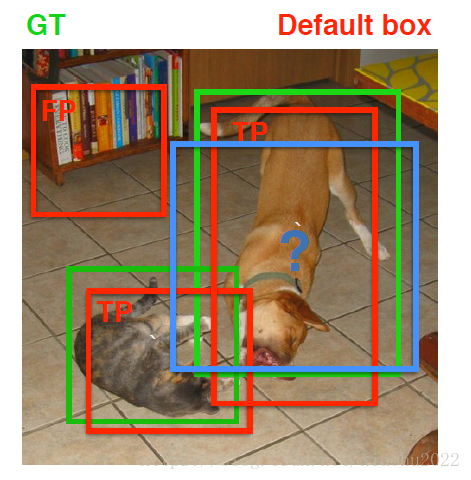# 1. 引言

• 引入了SSD，这是一种针对多个类别的单次检测器，比先前的先进的单次检测器（YOLO）更快，并且准确得多，事实上，与执行显式区域提出和池化的更慢的技术具有相同的精度（包括Faster R-CNN）。
• SSD的核心是预测固定的一系列默认边界框的类别分数和边界框偏移，使用更小的卷积滤波器应用到特征映射上。
• 为了实现高检测精度，我们根据不同尺度的特征映射生成不同尺度的预测，并通过纵横比明确分开预测。
• 这些设计功能使得即使在低分辨率输入图像上也能实现简单的端到端训练和高精度，从而进一步提高速度与精度之间的权衡。
• 实验包括在PASCAL VOC，COCO和ILSVRC上评估具有不同输入大小的模型的时间和精度分析，并与最近的一系列最新方法进行比较

# The Single Shot Detector (SSD)SSD framework:
-对于每个对象训练，SSD只需要一个输入图像和ground truth boxes。卷积的方式,我们评价一系列小的(例如:4)。不同aspect ratios的default boxes在几个特征图的每个位置，不同尺度(例如8×8和4×4 (b)和©)。对于每个each default box,我们预测shape offsets和confidence为所有对象类别( ( c 1 , c 2 ⋅ ⋅ ⋅ c p ) (c_1, c_2···c _p) )。在训练期间，我们首先把default boxes与ground truth boxes相匹配。

# 2.1 Model

SSD方法基于前馈卷积网络，该网络产生固定大小的边界框集合，并对这些边界框中存在的目标类别实例进行评分，然后进行非极大值抑制步骤来产生最终的检测结果。早期的网络层基于用于高质量图像分类的标准架构（在任何分类层之前被截断），我们将其称为基础网络。Multi-scale feature maps for detection

Convolutional predictors for detection

Default boxes and aspect ratios

SSD在不同的特征层中考虑不同的尺度，RPN在一个特征层考虑不同的尺度。

## 2.2 训练

Matching Strategy

• 对于图片中每个ground truth，找到与其IOU最大的先验框，该先验框与其匹配，这样，可以保证每个ground truth一定与某个先验框匹配。通常称与ground truth匹配的先验框为正样本，反之，若一个先验框没有与任何ground truth进行匹配，那么该先验框只能与背景匹配，就是负样本。一个图片中ground truth是非常少的， 而先验框却很多，如果仅按第一个原则匹配，很多先验框会是负样本，正负样本极其不平衡，所以需要第二个原则。
• 对于剩余的未匹配default box，若与某个ground truth的IOU大于某个阈值（一般是0.5），那么该default box也与这个ground truth进行匹配。这意味着某个ground truth可能与多个default box匹配。Training Objective
x i j p = { 1 , 0 } x_{ij}^p = \lbrace 1,0 \rbrace 是第 i i 个default box匹配到类别 p p 的第 j j 个实际ground truth， ∑ i x i j p ≥ 1 \sum_i x_{ij}^p \geq 1 ，总体目标损失函数是定位损失（loc）和置信度损失（conf）的加权和：
L ( x , c , l , g ) = 1 N ( L c o n f ( x , c ) + α L l o c ( x , l , g ) ) L(x, c, l, g) = \frac{1}{N}(L_{conf}(x, c) + \alpha L_{loc}(x, l, g))
N N 是匹配的默认边界框的数量。定位损失是 predicted box( l l )与ground truth box( g g )参数之间的Smooth L1损失。类似于Faster R-CNN，我们回归默认边界框( d d )的中心偏移量( c x , c y c_x, c_y )和其宽度( w w )、高度( h h )的偏移量。
L l o c ( x , l , g ) = ∑ i ∈ P o s N ∑ m ∈ { c x , c y , w , h } x i j k s m o o t h L 1 ( l i m − g ^ j m ) g ^ j c x = ( g j c x − d i c x ) / d i w g ^ j c y = ( g j c y − d i c y ) / d i h g ^ j w = log ⁡ ( g j w d i w ) g ^ j h = log ⁡ ( g j h d i h ) L_{loc}(x,l,g) = \sum_{i \in Pos}^N \sum_{m \in \lbrace cx, cy, w, h \rbrace} x_{ij}^k \mathtt{smooth}_{L1}(l_{i}^m - \hat{g}_j^m) \\ \hat{g}_j^{cx} = (g_j^{cx} - d_i^{cx}) / d_i^w \quad \quad \hat{g}_j^{cy} = (g_j^{cy} - d_i^{cy}) / d_i^h \\ \hat{g}_j^{w} = \log\Big(\frac{g_j^{w}}{d_i^w}\Big) \quad \quad \hat{g}_j^{h} = \log\Big(\frac{g_j^{h}}{d_i^h}\Big)L c o n f ( x , c ) = − ∑ i ∈ P o s N x i j p l o g ( c ^ i p ) − ∑ i ∈ N e g l o g ( c ^ i 0 ) w h e r e c ^ i p = exp ⁡ ( c i p ) ∑ p exp ⁡ ( c i p ) L_{conf}(x, c) = - \sum_{i\in Pos}^N x_{ij}^p log(\hat{c}_i^p) - \sum_{i\in Neg} log(\hat{c}_i^0)\quad \mathtt{where}\quad\hat{c}_i^p = \frac{\exp(c_i^p)}{\sum_p \exp(c_i^p)}

Choosing Scales and Aspect Ratios for Default Boxes

s k = s min + s max − s min m − 1 ( k − 1 ) , k ∈ [ 1 , m ] s_k = s_\text{min} + \frac{s_\text{max} - s_\text{min}}{m - 1} (k - 1),\quad k\in [1, m] 其中 s min s_\text{min} 为0.2， s max s_\text{max} 为0.9

Hard Negative Mining
ground truth匹配的先验框为正样本，反之，若一个先验框没有与任何ground truth进行匹配，那么该先验框只能与背景匹配，就是负样本。尽管一个ground truth可以与多个先验框匹配，但是ground truth相对先验框还是太少了，经过matching后，很多default box是负样本，这将导致正样本、负样本不均衡，训练难以收敛。因此，该论文将负样本根据置信度进行排序，选取误差的较大的top-k作为训练的负样本，保证负样本、正样本的比例为3：1。

Data Augmentation

• 使用整个原始输入图像。
• 采样一个图像块，使得与目标之间的最小Jaccard重叠为0.1，0.3，0.5，0.7或0.9。
• 随机采样一个图像块。

09-202万+01-038203
02-21363
01-048996
10-064154
11-29134
11-077179
09-27104
08-1368
02-06326
09-2240
03-11183
10-062492
06-242071
10-14166
06-158560
©️2020 CSDN 皮肤主题: 编程工作室 设计师:CSDN官方博客